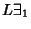Kreisel Conjecture

A Conjecture in Decidability theory which postulates that, if there is a uniform bound to the lengths of shortest proofs of instances of, then the universal generalization is necessarily provable in Peano Arithmetic. The Conjecture was proven true by M. Baaz in 1988 (Baaz and Pudlák 1993).

References

Baaz, M. and Pudlák P. ``Kreisel's Conjecture for. In Arithmetic, Proof Theory, and Computational Complexity, Papers from the Conference Held in Prague, July 2-5, 1991 (Ed. P. Clote and J. Krajicek). New York: Oxford University Press, pp. 30-60, 1993.

Dawson, J. ``The Gödel Incompleteness Theorem from a Length of Proof Perspective.'' Amer. Math. Monthly 86, 740-747, 1979.

Kreisel, G. ``On the Interpretation of Nonfinitistic Proofs, II.'' J. Symbolic Logic 17, 43-58, 1952.